Molecular Equation, Ionic Equation, Net Ionic EquationIn my previous post we learn about chemical Equation and the types of chemical reactions.  All cited equations are example of molecular equation.  Molecular equations show the reaction of molecules where all species are present which exist as whole unit or molecules, as shown in the example below: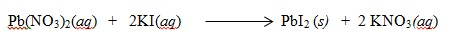The equation above is an example of molecular equation and a double replacement reaction, It shows the reaction of lead nitrate molecule in reaction with potassium iodide forming lead  iodide precipitate and potassium nitrate molecules.  Although the equation gives all the required information of the reactants and products, it does not show what happens to the substances in solution.

Ionic equation on the other hand is a kind of equation which shows what happens to the substances in solution.  It shows dissolve species as free ions. See the example below:This shows what happens to the reaction of lead nitrate  and potassium iodide in solution.  If you will notice all reactants and products are dissociated in water except lead iodide.  This is because lead iodide is not soluble in water.  Now in predicting if precipitate will be formed in the reaction, refer to the solubility rules.  Once the solute is soluble it should be written in ionized form as it shown in the example above and if not soluble it should be written as a whole molecule.

Now to determine the Net Ionic Equation, spectator ions are being canceled out or eliminated.  Spectator ions are ions which are not included in the overall reaction  These are ions that are present both in reactants side and in the products side.  After the spectator ions are eliminated, what will be left is the net ionic equation.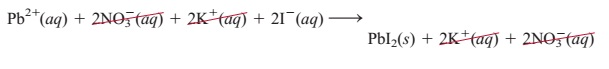After all spectator ions are eliminated the net ionic equation for the above reaction will be: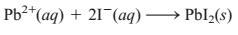Net ionic equation shows only the species that take part in the reaction.

In summary, there are several rules to follow in order to come up with a net ionic equation:

1.  A reaction must be written in molecular form specifying the reactants and products if soluble or not in water.  If soluble in water aq is used which means that the substance is dissociated in water, if not soluble, s is written as subscript which means that precipitate is formed.   And the equation must be balanced.

2.  Write the ionic equation.  Substances that are soluble in water must be written in ionized form while the substance which is not soluble in water should be written as a whole molecule.

3.  Cancel all the spectator ions both in the reactants and products side.  Then write the net ionic equation.

4.  Finalize by checking the charges and number of atoms in the net ionic equation.

1.  Predict what happens when a potassium phosphate (K3PO4) solution is mixed with calcium nitrate
[Ca(NO3)2] solution. Write the net ionic equation for this reaction.

Step 1.  Write the balanced equation of the reaction.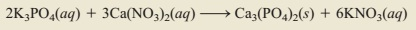Step 2.  Write the ionic equation by writing the soluble substances in ionized form.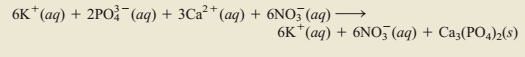Step 3.  Cancel all the spectator ions and write the net ionic equation: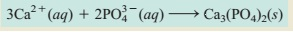Step 4.  Check if the net ionic equation has balance charges and number of atoms
Reactants,      +6 and -6 = 0    ,   Products  = 0

TRY THIS:

Write the balanced molecular equation, ionic equation and net ionic equation of the following:

1.  Na2S(aq)   +     ZnCl2(aq)  >>>

2.  K3PO4(aq)   +   Sr(NO3)2(aq)  >>>

3.  Mg(NO3)2 (aq)  +   NaOH(aq)  >>>>

This post first appeared on Science Concepts And Questions (K To 12), please read the originial post: here

Share the post

Molecular Equation, Ionic Equation, Net Ionic Equation

×

Subscribe to Science Concepts And Questions (k To 12)

Get updates delivered right to your inbox!

×